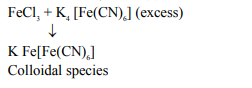# Acidic ferric chloride solution on treatment with excess of potassium ferrocyanide gives a Prussian blue coloured colloidal species. It is :Question:

Acidic ferric chloride solution on treatment with excess of potassium ferrocyanide gives a Prussian blue coloured colloidal species. It is :

1. $\mathrm{Fe}_{4}\left[\mathrm{Fe}(\mathrm{CN})_{6}\right]_{3}$

2. $\mathrm{K}_{5} \mathrm{Fe}\left[\mathrm{Fe}(\mathrm{CN})_{6}\right]_{2}$

3. $\mathrm{HFe}\left[\mathrm{Fe}(\mathrm{CN})_{6}\right]$

4. $\mathrm{KFe}\left[\mathrm{Fe}(\mathrm{CN})_{6}\right]$

Correct Option: , 4

Solution: